# Using Channel Ops with GIMP

Channel ops are one of the most powerful and simple tools available to you with the GIMP. Here's a brief example of what each channel op is, and what it's used for.
[ Add | Subtract | Difference | Darker | Lighter | Composite | Blend | Multiply | Interpolate/Extrapolate ]

For further reference, also see Quartic's Explanation and Kai's Power Tips.

## Introduction

Channel ops are operations that typically involve two images. Each channel operation compares and does some sort of manipulation based on the pixels in two images. Some channel ops have intuitive operations, and some have fairly obscure operation, but they all are pretty easy to grasp, and they have very powerful uses. Note: channel ops can only operate on images that are exactly the same size. Even a pixel off will screw it up! With that in mind...

Add takes two images, and adds the RGB or greyscale values of the pixels. For 8 bit greyscale/24 bit color, there is a threshold of 255.

What does this mean? Well, say for example you have a completely white image. All the values, therefore, are 255. When you Add this to another image, it will turn the entire image completely white as well, because 255 + n >= 255. (Negative values not accepted of course :).

If you have a completely black canvas, all the values are zero. When you Add this to another image, it has no effect, since 0 + n = n, no matter what.

How is this useful? If you Add an image with a black and white pattern on it, the white areas effectively blank out the other image, while the black areas leave the other image untouched. A picture is worth a thousand words, so take a look at this:Add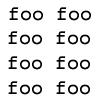results in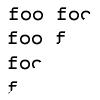Also, you don't have to use just black and white images...here's an Add using a vertical fade for one of the images: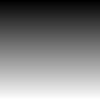Add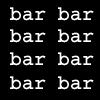results inNote that you can also do this with full color images. If you do, it takes values from each channel individually when doing the calculations.

## Subtract

As its name implies, Subtract subtracts the second image's values from the first's. Unlike Add, Subtract produces different results when you change the order of the images. You've probably made the intuitive leap here: like you learned in elementary arithmetic, addition is commutative and subtraction is not.

This means that if a white image is subtracted from any image, it results in a black image. A black image subtracted from an image has no effect. White areas in a subtracting image produce complete black, and black areas in a subtracting image have no effect whatsoever.

The key thought here is, you're doing math with pixels. It's not some magic filter that does some whiz-bang transformation based on the sine of the hypotenuse of something (if it was, I'd never understand it, I was terrible at trigonometry). You're doing some very simple mathematics.

A picture is worth a thousand words:Subtractresults in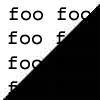And it follows that:Subtractresults in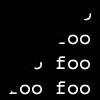## Difference

Difference is a very simple channel operation. It outputs the difference between two images, or the absolute value (or magnitude) of one image subtracted from the other. Difference is commutative.

That means, values that are similar in each image get very dark (or black), and values that are different in each image get very bright (or white, as the case may be). Areas of maximum and minimum difference result in white and black pixels, respectively. (Please note that when I say black and white, it's relative to the channel you're working on...white in the red channel becomes bright red, etc.)Differenceresults in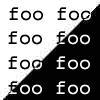This also leads to an interesting discovery:Differenceresults in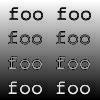## Lighter

This is a channel operation that I wrote because Photoshop has it, and I wanted to use it. Lighter compares two images and results in the lightest values from each image. This chanop is quite simple, so here's a quick visual example:Lighterresults in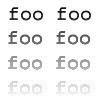## Darker

This channel operation compares two images and results in the darkest values from each image. For slightly more info, see
Lighter.Darkerresults in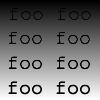## Composite

This is one of my favorite and most useful channel operations. This channel operation is fairly unique in that it uses THREE values: two source images and one mask image. Here's how it works:

If a pixel is dark in the mask, it outputs the values from the first image. If the pixel is bright in the mask, it outputs values from the second image. The upshot of all this is, black pixels get filled in with the first image, and white pixels get filled in with the second image. If a pixel is grey in the mask, it basically blends the two images together.

This is really incredibly handy, and most of my fun tips do something with Composite. Here are some of the applications of Composite, using my stock images:MaskImage 1Image 2 results in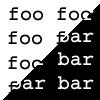And here's another good example:MaskImage 1Image 2 results in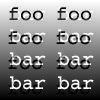Important: The mask's pixels never become part of the resulting image; they just define how much gets taken from which image.

## Blend

This channel operation averages the two images together. This is an adjustable average; you can weight it towards one image or another. Since this is another fairly straightforward and easy-to-understand chanop, I'm going to skip straight to the pictures:Blendresults in(Are you getting sick of me using the same images each time? Well, try using some of your own! Experiment!)

## Multiply

Well, I didn't really understand how this worked, so I'll fill in Quartic's elegant explanation along with my own commentary:

```From: Federico Mena Quintero

Multiply - Multiplies the pixels of one image by the pixels of the
other one.  If the pixels were in the real range [0, 1], it could be
viewed as

for (every pixel)
dest_pixel = source1_pixel * source2_pixel;

And this would also produce values in the range [0, 1].  However, our
integer values are in [0, 255], so the actual formula used is

dest_pixel = source1_pixel * source2_pixel / 255;

This is to 'bring back' the values to [0, 255].  Note that multiplying
an image by another one always darkens the result.  This is because a
number in the range [0, 1] multiplied by another number in the same
range will always be smaller than or equal to either of them.
```
Basically, this treats both images as being of "unit brightness" (I think that's the right term), in other words, with a maximum brightness of one and a minimum brightness of zero. It then multiplies the unit brightness of each image together. Since multiplying anything less than 1 by something else always results in a smaller value, the image always gets darker.Multiplyresults in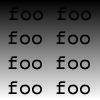Top
Tips Tricks Tutorials